## 25 Questions MCQ Test Mathematics (Maths) Class 9 | Test: Quadrilaterals- 2

Description
This mock test of Test: Quadrilaterals- 2 for Class 9 helps you for every Class 9 entrance exam. This contains 25 Multiple Choice Questions for Class 9 Test: Quadrilaterals- 2 (mcq) to study with solutions a complete question bank. The solved questions answers in this Test: Quadrilaterals- 2 quiz give you a good mix of easy questions and tough questions. Class 9 students definitely take this Test: Quadrilaterals- 2 exercise for a better result in the exam. You can find other Test: Quadrilaterals- 2 extra questions, long questions & short questions for Class 9 on EduRev as well by searching above.
QUESTION: 1

Solution:
QUESTION: 2

Solution:
QUESTION: 3

### D and E are the mid-points of the sides AB and AC res. Of △ABC. DE is produced to F. To prove that CF is equal and parallel to DA, we need an additional information which is:

Solution:
QUESTION: 4

P, Q, R are the mid- points of AB, BC, AC res, If AB = 10cm, BC = 8cm, AC = 12cm, Find the perimeter of △PQR.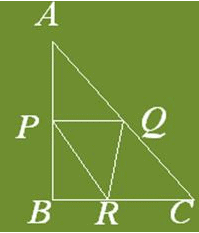Solution:
QUESTION: 5

In Triangle ABC which is right angled at B. Given that AB = 9cm, AC = 15cm and D, E are the mid-points of the sides AB and AC res. Find the length of BC?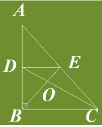Solution:
QUESTION: 6

Three Statements are given below:
(I) In a, Parallelogram the angle bisectors of 2 adjacent angles enclose a right angle.
(II) The angle bisector of a Parallelogram form a Rectangle.
(III) The Triangle formed by joining the mid-points of the sides of an isosceles triangle is not necessarily an isosceles triangle. Which is True?

Solution:
QUESTION: 7

If APB and CQD are 2 parallel lines, then the bisectors of the angles APQ, BPQ, CQP and PQD form, square only if

Solution:
Line APB is parallel to CQD
when we join PQ it will be transversal
then angleBPQ=angleCQP    (alternate angles)
angleAPQ=anglePQD
when we will draw bisectors
then the figure formed will have opposite angles equal
which means that it is a parallelogram

QUESTION: 8

If bisectors of ∠A and ∠B of a quadrilateral ABCD intersect each other at P, of ∠B and ∠C at Q, of ∠C and ∠D at R and of ∠D and ∠A at S, then PQRS is a

Solution:

To show: ∠PSR + ∠PQR = 180°
∠SPQ + ∠SRQ = 180°

In △DSA,

∠DAS + ∠ADS + ∠DSA = 180° (angle sum property)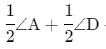+ ∠ SA = 180° (since RD and AP are bisectors of ∠D and ∠A)

∠DSA = 180°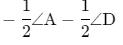∠PSR = 180°−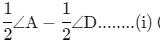(∵ ∠DSA = ∠PSR are vertically opposite angles)

Similarly,

∠PQR = 180°−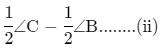Adding (i) and (ii), we get, ∠PSR + ∠PQR = 180°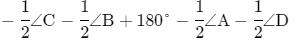=360° − 1/2 ​× (∠A + ∠B + ∠C + ∠D)

=360°− 1/2​ × 360° = 180° ∴ ∠PSR + ∠PQR = 180°

∠SPQ + ∠SRQ + ∠PSR + ∠PQR = 360°
=> ∠SPQ + ∠SRQ + 180° = 360°
=> ∠SPQ + ∠SRQ = 180°

Hence, showed that opposite angles of PQRS are supplementary.

QUESTION: 9

The Diagonals AC and BD of a Parallelogram ABCD intersect each other at the point O such that ∠DAC = 30 and ∠AOB = 70. Then, ∠DBC?

Solution:
QUESTION: 10

In Parallelogram ABCD, bisectors of angles A and B intersect each other at O. The measure of ∠AOB is

Solution:
QUESTION: 11

Three statements are given below:
(I) In a Rectangle ABCD, the diagonals AC bisects ∠A as well as ∠C.
(II) In a Square ABCD, the diagonals AC bisects ∠A as well as ∠C.
(III) In rhombus ABCD, the diagonals AC bisects ∠Aas well as ∠C.
Which is True?

Solution:
QUESTION: 12

D and E are the mid-points of the sides AB and AC of △ABC and O is any point on the side BC, O is joined to A. If P and Q are the mid-points of OB and OC res, Then DEQP is

Solution:
QUESTION: 13

Given Rectangle ABCD and P, Q, R and S are the mid-points of the sides AB, BC, CD and DA res. If length of a diagonal of Rectangle is 8cm, then the quadrilateral PQRS is a

Solution:
QUESTION: 14

In the given figure, ABCD is a Rhombus. Then,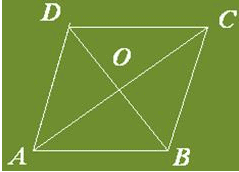Solution:
QUESTION: 15

D and E are the mid-points of the sides AB and AC. Of △ABC. If BC = 5.6cm, find DE.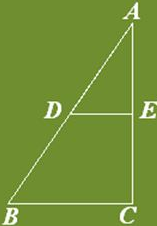Solution:
QUESTION: 16

In a triangle P, Q and R are the mid-points of the sides BC, CA and AB res. If AC = 21cm, BC = 29cm and AB = 30cm, find the perimeter of the quadrilateral ARPQ?

Solution:
QUESTION: 17

The bisectors of the angles of a Parallelogram enclose a

Solution:
QUESTION: 18

Opposite angles of a Quadrilateral ABCD are equal. If AB = 4cm, find the length of CD.

Solution:
QUESTION: 19

In a Trapezium ABCD, if AB ║ CD, then (AC2+ BD2) = ?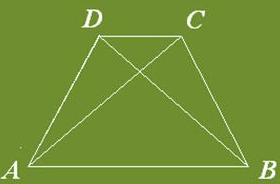Solution:
QUESTION: 20

In quadrilateral ABCD, ∠B=90, ∠C−∠D = 60 and ∠A−∠C−∠D = 10. Find ∠A, ∠C and ∠D.

Solution:
QUESTION: 21

If a Quadrilateral ABCD,∠A = 90 and AB = BC = CD = DA, Then ABCD is a

Solution:
QUESTION: 22

Solution:
QUESTION: 23

In △ABC, EF is the line segment joining the mid-points of the sides AB and AC. BC = 7.2cm, Find EF.

Solution:
QUESTION: 24

In the figure, ABCD is a rhombus, whose diagonals meet at 0. Find the values of x and y.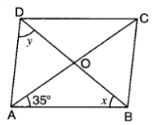Solution:

Since diagonals of a rhombus bisect each other at right angle .

∴ In △AOB , we have

∠OAB + ∠x + 90° = 180°

∠x = 180° -  90° - 35° [∵ ∠OAB = 35°]

= 55°

Also, ∠DAO = ∠BAO = 35°

∴ ∠y + ∠DAO + ∠BAO + ∠x = 180°

⇒ ∠y + 35° + 35° + 55° =  180°

⇒ ∠y = 180° - 125° = 55°

Hence the values of x and y are x =  55°, y =  55°.

QUESTION: 25

The diagonals AC and BD of a parallelogram ABCD intersect each other at the point O. If ∠DAC = 32 and ∠AOB = 70 then, ∠DBC is equal to

Solution: